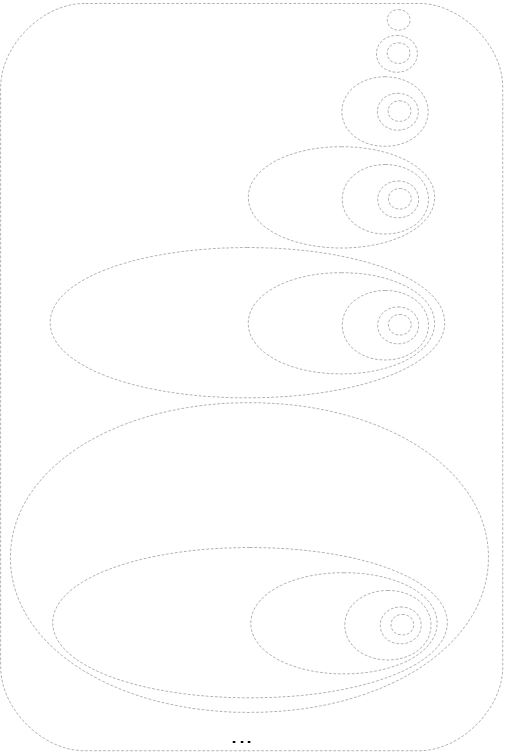Welcome guest
You're not logged in.
245 users online, thereof 0 logged in

## Definition: Set-theoretic Definitions of Natural Numbers (Ernst Zermelo 1908, John von Neumann 1923)

The set of natural numbers $$\mathbb N$$ is defined using the concept ordinals, as follows:

### Definition due to von Neumann (1923)

(1) The empty set (as the first ordinal)1 represents the first natural number:

$0:=\{\emptyset\}.$(2) Once we have the ordinal $$n=\alpha$$, we can construct a bigger ordinal2 using recursively the formula for constructing successors of ordinals, denoting the successor $$n^+$$ of the natural number $$n$$:
$n^+:=s(\alpha):=\alpha\cup\{\alpha\}=n\cup \{n\}.$
Applying the set axioms and this construction systematically, it gives us a chain of ordered ordinals

$\begin{array}{rcl}0&:=&\emptyset,\\1&:=&0\cup\{0\}=\emptyset\cup\{\emptyset\}=\{\emptyset\},\\2&:=&1\cup\{1\}=\{\emptyset\}\cup\{\{\emptyset\}\}=\{\emptyset,\{\emptyset\}\},\\3&:=&2\cup\{2\}=\{\emptyset,\{\emptyset\}\}\cup\{\{\emptyset,\{\emptyset\}\}\}=\{\emptyset,\{\emptyset\},\{\emptyset,\{\emptyset\}\}\},\\&\vdots&\\n^+&:=&n\cup\{n\},\\&\vdots&\end{array}$

which can be visualized in the following figureand for which we introduce the notation $$0,1,2,3,\ldots$$:

$0 < 1 < 2 < 3 < \ldots$

Due to the axiom of infinity we can postulate the existence of an infinite set, which is “contains” all such sets.3

$\mathbb N:=\bigcup n=\{0,1,2,3,\ldots.\}$

1 Please note that it is well defined due to the axiom of existence of empty set.

2 Ordinals are sets with some interesting properties, including trichotomy, ensuring that all ordinals can be compared with each other by the relation
$\alpha < \beta:\Leftrightarrow \alpha\in\beta.$
For any two ordinals, and in particular for natural numbers, we can therefore always decide which one is “bigger”, “smaller”, or whether they are equal to each other.

3 Please note that this infinite set is an ordinal by definition. However, we have not built by the above construction formula, i.e. it is not a successor of any “previous” ordinal. In other words, $$\mathbb N$$ is the first limit ordinal.

### Definition due to Ernst Zermelo (1908)

The set $$\mathbb N$$ of natural numbers is defined recursively by: $\begin{array}{rcl}0&:=&\emptyset,\\1&:=&\{0\}=\{\emptyset\},\\2&:=&\{1\}=\{\{\emptyset\}\},\\3&:=&\{2\}=\{\{\{\emptyset\}\}\},\\&\vdots&\\n^+&:=&\{n\}=\underbrace{\{\ldots\{ }_{n+1\text{ times}}\emptyset\underbrace{\}\ldots\} }_{n+1\text{ times}},\\&\vdots&\\\end{array}$

This definition can be visualized as follows: